ICSE Class 10 Mole Concept Solution New

## ICSE Class 10 Mole Concept Solution By Clarify knowledge

ICSE Class 10 Mole Concept Solution New Pattern

## Best Reference Books For Class 10 ICSE

Best Reference Books For Class 10 ICSE Physics Is OSWAL GURUKUL

## Chapter 5 - Mole Concept And Stoichiometry Exercise Ex. 5A

Question 1

State :

(a) Gay-Lussac's Law of combining volumes.

(a) Gay-Lussac's law states that when gases react, they do so in volumes which bear a simple ratio to one another, and to the volume of the gaseous product, provided that all the volumes are measured at the same temperature and pressure.

(b) Avogadro's law states that equal volumes of all gases under similar conditions of temperature and pressure contain the same number of molecules.Question 2

(a) What do you mean by stoichiometry?

(b) Define atomicity of a gas. State the atomicity of Hydrogen, Phosphorus and Sulphur.

(c) Differentiate between N2 and 2N.Solution 2

(a) stoichiometry measures quantitative relationships and is used to determine the amount of products/reactants that are produced/needed in a given reaction.

(b) The number of atoms in a molecule of an element is called its atomicity. Atomicity of Hydrogen is 2, phosphorus is 4 and sulphur is 8.

(c) Nmeans 1 molecule of nitrogen and 2N means two atoms of nitrogen.

N2 can exist independently but 2N cannot exist independently.Question 3

Explain Why?

(a) "The number of atoms in a certain volume of hydrogen is twice the number of atoms in the same volume of helium at the same temperature and pressure."

(b) "When stating the volume of a gas, the pressure and temperature should also be given."

(c)Inflating a balloon seems to violate Boyle's law.Solution 3

(a) This is due to Avogadros Law which states Equal volumes of all gases under similar conditions of temperature and pressure contain the same number of molecules.

Now volume of hydrogen gas =volume of helium gas

n molecules of hydrogen =n molecules of helium gas

nH2=nHe

1 mol. of hydrogen has 2 atoms of hydrogen and I molecule of helium has 1 atom of helium

Therefore 2H=He

Therefore atoms in hydrogen is double the atoms of helium.

(b) For a given volume of gas under given temperature and pressure, a change in any one of the variable i.e., pressure or temperature changes the volume.

(c) Inflating a balloon seems violating Boyles law as volume is increasing with increase in pressure. Since the mass of gas is also increasing.Question 4

(a) Calculate the volume of oxygen at S.T.P required for the complete combustion of 100 litres of carbon monoxide at the same temperature and pressure.

2CO + O22CO2

(b) 200 cm3 of hydrogen and 150 cm3 of oxygen are mixed and ignited, as per the following reaction,

2H+ O2  2H2O

What volume of oxygen remains unreacted?Solution 4

(a)2CO + O22CO2

2 V    1 V     2 V

2 V of CO requires = 1V of O2

so, 100 litres of CO requires = 50 litres of O2

(b) 2H+ O22H2O

2 V         1V            2V

From the equation, 2V of hydrogen reacts with 1V of oxygen

so 200cm3 of Hydrogen reacts with = 200/2= 100 cm3

Hence, the unreacted oxygen is 150 - 100 = 50cmof oxygen.Question 5

24 cc Marsh gas (CH4) was mixed with 106 cc oxygen and then exploded. On cooling the volume of the mixture became 82 cc, of which, 58 cc was unchanged oxygen. Which law does this experiment support? Explain with calculation.Solution 5

This experiment supports Gay lussac's law of combining volumes.

Since the unchanged or remaining O2 is 58 cc so, used oxygen 106 - 58 = 48cc

According to Gay lussac's law, the volumes of gases reacting should be in a simple ratio.

CH4+2O2CO2 + 2H2O

1 V2 V

24 cc48 cc

i.e. methane and oxygen react in a 1:2 ratio.Question 6

What volume of oxygen would be required to burn completely 400 ml of acetylene [C2H2]? Also calculate the volume of carbon dioxide formed.

2C2H2 + 5O4CO2 + 2H2O (l)Solution 6

2C2H2 + 5O4CO2 + 2H2O (l)

2 V 5 V 4 V

From equation, 2 V of C2Hrequires = 5 V of O2

So, for 400ml C2H, O2 required = 400 5/2 =1000 ml

Similarly, 2 V of C2H2 gives = 4 V of CO2

So, 400ml of C2H2 gives CO2 = 400 4/2 = 800mlQuestion 7

112 cm3 of H2S(g) is mixed with 120 cm3 of Cl2(g) at STP to produce HCl(g) and sulphur(s). Write a balanced equation for this reaction and calculate (i) the volume of gaseous product formed (ii) composition of the resulting mixture.Solution 7

Balanced chemical equation:

(i)At STP, 1 mole gas occupies 22.4 L.

As 1 mole H2S gas produces 2 moles HCl gas,

22.4 L H2S gas produces 22.4 × 2 = 44.8 L HCl gas.

Hence, 112 cm3 H2S gas will produce 112 × 2 = 224 cm3 HCl gas.

(ii) 1 mole H2S gas consumes 1 mole Cl2 gas.

This means 22.4 L H2S gas consumes 22.4 L Cl2 gas at STP.

Hence, 112 cm3 H2S gas consumes 112 cm3 Cl2 gas.

120 cm3 - 112 cm= 8 cmCl2 gas remains unreacted.

Thus, the composition of the resulting mixture is 224 cm3HCl gas + 8 cm3 Cl2 gas.Question 8

1250cc of oxygen was burnt with 300cc of ethane [C2H6]. Calculate the volume of unused oxygen and the volume of carbon dioxide formed:

Solution 8

From the equation, 2V of ethane reacts with 7V oxygen.

So, 300 cc of ethane reacts withHence, unused O2 = 1250 - 1050 = 200 cc

From 2V of ethane, 4V of CO2 is produced.

So, 300 cc of ethane will produceQuestion 9

What volume of oxygen at STP is required to affect the combustion of 11 litres of ethylene [C2H4] at 273o C and 380 mm of Hg pressure?

C2H4+3O22CO2 + 2H2OSolution 9

C2H4+3O22CO2 + 2H2O

1V      3V

11litre   33 litre

Question 10

Calulate the volume of HCl gas formed and chlorine gas required when 40 ml of methane reacts completely with chlorine at S.T.P.

CH4 + 2Cl2 CH2Cl2+2HClSolution 10

CH4 + 2Cl2 CH2Cl+2HCl

1 V 2 V 1 V 2 V

From equation, 1V of CH4 gives = 2 V HCl

so, 40 ml of methane gives = 80 ml HCl

For 1V of methane = 2V of Cl2 required

So, for 40ml of methane = 40 2 = 80 ml of Cl2Question 11

What volume of propane is burnt for every 500 cm3 of air used in the reaction under the same conditions? (assuming oxygen is 1/5th of air)

C3H+ 5O2 3CO2 + 4H2OSolution 11

C3H+ 5O2 3CO2 + 4H2O

1 V 5 V 3 V

From equation, 5 V of O2 required = 1V of propane

so, 100 cm3 of Owill require = 20 cm3 of propaneQuestion 12

450 cm3 of nitrogen monoxide and 200 cm3 of oxygen are mixed together and ignited. Caclulate the composition of resulting mixture.

2NO + O2 2NO2Solution 12

2NO + O2 2NO2

2 V 1 V 2 V

From equation, 1V of O2 reacts with = 2 V of NO

200cm3 oxygen will react with = 200 2 =400 cmNO

Hence, remaining NO is 450 - 400 = 50 cm3

NO2 produced = 400cm3 because 1V oxygen gives 2 V NO2

Total mixture = 400 + 50 = 450 cm3Question 13

If 6 liters of hydrogen and 4 liters of chlorine are mixed and exploded and if water is added to the gases formed, find the volume of the residual gas.Solution 13

i. 6 litres of hydrogen and 4 litres of chlorine when mixed, results in the formation of 8 litres of HCl gas.

ii. When water is added to it, it results in the formation of hydrochloric acid. Chlorine acts as a limiting agent leving behind only 2 litres of hydrogen gas.

iii. Therefore, the volume of the residual gas will be 2 litres.Question 14

Ammonia may be oxidised to nitrogen monoxide in the presence of a catalyst according to the following equation.

4NH3 + 5O2 4NO + 6H2O

If 27 litres of reactants are consumed, what volume of nitrogen monoxide is produced at the same temperature and pressure?Solution 14

4NH3 + 5O2 4NO + 6H2O

4 V 5 V 4 V

9 litres of reactants gives 4 litres of NO

So, 27 litres of reactants will give = 27 4/9 = 12 litres of NOQuestion 15

A mixture of hydrogen and chlorine occupying 36 cm3 was exploded. On shaking it with water, 4cmof hydrogen was left behind. Find the composition of the mixture.Solution 15

H2 + Cl2HCl

1V 1V 2 V

Since 1 V hydrogen requires 1 V of oxygen and 4cm3 of H2 remained behind so the mixture had composition: 16 cm3 hydrogen and 16 cmchlorine.

Therefore Resulting mixture is H2 =4cm3,HCl=32cm3Question 16

What volume of air (containing 20% O2 by volume) will be required to burn completely 10 cm3 each of methane and acetylene?

CH4 + 2OCO2 + 2H2O

2C2H2 + 5O2 4CO2 + 2H2OSolution 16

CH4 + 2OCO2 + 2H2O

1 V 2 V 1 V

2C2H2 + 5O2 4CO2 + 2H2O

2 V 5 V 4 V

From the equations, we can see that

1V CH4 requires oxygen = 2 V O2

So, 10cm3 CH4 will require =20 cm3 O2

Similarly 2 V C2H2 requires = 5 V O2

So, 10 cm3 C2Hwill require = 25 cm3 O2

Now, 20 V O2 will be present in 100 V air and 25 V O2 will be present in 125 V air ,so the volume of air required is 225cm3Question 17

LPG has 60% propane and 40% butane: 10 litres of this mixture is burnt. Calculate the volume of carbon dioxide added to atmosphere.

C3H8 + 5O2 3CO2 + 4H2O

2C4H10 + 13O2 8CO2 + 10H2OSolution 17

C3H8 + 5O2 3CO2 + 4H2O

2C4H10 + 13O2 8CO2 + 10H2O

60 ml of propane (C3H8) gives 3 60 = 180 ml CO2

40 ml of butane (C4H10) gives = 8 40/2 = 160 ml of CO2

Total carbon dioxide produced = 340 ml

So, when 10 litres of the mixture is burnt = 34 litres of COis produced.Question 18

200 cm3 of CO2 is collected at S.T.P when a mixture of acetylene and oxygen is ignited. Calculate the volume of acetylene and oxygen at S.T.P. in original mixture.

2C2H2(g) + 5O2(g) 4CO2(g)+ 2H2O(g)Solution 18

2C2H2(g) + 5O2(g) 4CO2(g)+ 2H2O(g)

4 V CO2 is collected with 2 V C2H2

So, 200cm3 COwill be collected with = 100cm3 C2H2

Similarly, 4V of CO2 is produced by 5 V of O2

So, 200cm3 COwill be produced by = 250 ml of O2Question 19

You have collected (a) 2 litres of CO2 (b) 3 litres of chlorine (c) 5 litres of hydrogen (d) 4 litres of nitrogen and (e) 1 litres of SO2, under similar conditions of temperature and pressure. Which gas sample will have :

(a) the greatest number of molecules, and

(b) The least number of molecules?

According to Avogadro's law, equal volumes of gases contain equal no. of molecules under similar conditions of temperature and pressure. This means more volume will contain more molecules and least volume will contain least molecules.

So,

(a) 5 litres of hydrogen has greatest no. of molecules with the maximum volume.

(b) 1 litre of SO2 contains the least number of molecules since it has the smallest volume.Question 20

The gases chlorine, nitrogen, ammonia and sulphur dioxide are collected under the same conditions of temperature and pressure. The following table gives the volumes of gases collected and the number of molecules (x) in 20 litres of nitrogen. You are to complete the table giving the number of molecules in the other gases in terms of x.

Solution 20

Question 21

(i) If 150 cc of gas A contains X molecules, how many molecules of gas B will be present in 75 cc of B?

The gases A and B are under the same conditions of temperature and pressure.

(ii) Name the law on which the above problem is based.Solution 21

(i) According to Avogadro's law, under the same conditions of temperature and pressure, equal volumes of different gases have the same number of molecules.

As 150 cc of gas A contains X molecules, 150 cc of gas B also contains X molecules.

So, 75 cc of B will contain X/2 molecules.

(ii) The problem is based on Avogadro's law.

## Chapter 5 - Mole Concept And Stoichiometry Exercise Ex. 5B

Question 1

(a) The relative atomic mass of Cl atom is 35.5 a.m.u. Explain this statement.

(b) What is the value of Avogadro's number?

(c) What is the value of molar volume of a gas at S.T.P?Solution 1

a) This statement means one atom of chlorine is 35.5 times heavier than 1/12 time of the mass of an atom C-12.

b)The value of avogadro's number is 6.023 1023

c) The molar volume of a gas at STP is 22.4 dm3 at STPQuestion 2

Define or explain the terms (a) Vapour density

(b) Molar volume

(c) Relative atomic mass

(d) Relative molecular mass

(f) Gram atom

(g) MoleSolution 2

(a) The vapour density is the ratio between the masses of equal volumes of gas and hydrogen under the conditions of standard temperature and pressure.

(b) Molar volume is the volume occupied by one mole of the gas at STP. It is equal to 22.4 dm3.

(c) The relative atomic mass of an element is the number of times one atom of the element is heavier than 1/12 times of the mass of an atom of carbon-12.

(d) The relative molecular mass of an compound is the number that represents how many times one moleculae of the substance is heavier than 1/12 of the mass of an atom of carbon-12.

(e) The number of atoms present in 12g (gram atomic mass) of C-12 isotope, i.e. 6.023 x1023 atoms.

(f) The quantity of the element which weighs equal to its gram atomic mass is called one gram atom of that element.

(g) Mole is the amount of a substance containing elementary particles like atoms, molecules or ions in 12 g of carbon-12.Question 3

(a) What are the main applications of Avogadro's Law?

(b) How dose Avogadro's Law explain Gay-Lussac's Law of combining volumes?Solution 3

(a) Applications of Avogadro's Law :

(1) It explains Gay-Lussac's law.

(2) It determines atomicity of the gases.

(3) It determines the molecular formula of a gas.

(4) It determines the relation between molecular mass and vapour density.

(5) It gives the relationship between gram molecular mass and gram molecular volume.

(b) According to Avogadro's law under the same conditions of temperature and pressure, equal volumes of different gases have the same number of molecules.

Since substances react in simple ratio by number of molecules, volumes of the gaseous reactants and products will also bear a simple ratio to one another.This what Gay Lussac's Law says.

H2 + Cl2 ? 2HCl

1V 1V 2V(By Gay-Lussacs law)

n molecules n molecules 2n molecules (By Avogadros law)Question 4

Calculate the relative molecular masses of:

(a) Ammonium chloroplatinate, (NH4)2 PtCl6

(b) Potassium chlorate (c) CuSO4. 5H2O (d) (NH4)2SO4

(e) CH3COONa (f) CHCl3 (g) (NH4)2 Cr2O7Solution 4

(a) (2N)28 + (8H)8 + (Pt)195 + (6Cl)35.5 x 6 = 444

(b) KClO3 = (K)39 + (Cl)35.5 + (3O)48 = 122.5

(c) (Cu)63.5 + (S)32 + (4O)64 + (5H2O)5 x 18 = 249.5

(d) (2N)28 + (8H)8 + (S)32 + (4O)64 = 132

(e) (C)12 + (3H)3 + (C)12 + (2O)32 + (Na)23 = 82

(f) (C)12 + (H)1+ (3Cl)3 x 35.5 = 119.5

(g) (2N)28 + (8H)8 + (2Cr)2 x 51.9+ (7O)7 x 16 = 252Question 5

Find the

(a) number of molecules in 73 g of HCl,

(b) weight of 0.5 mole of O2,

(c) number of molecules in 1.8 g of H2O

(d) number of moles in 10 g of CaCO3

(e) Weight of 0.2 mole of H2 gas,

(f) Number of molecules in 3.2 g of SO2.Solution 5

(a) No. of molecules in 73 g HCl = 6.023 x1023 x 73/36.5(mol.

mass of HCl)

= 12.04 x 1023

(b) Weight of 0.5 mole of O2 is = 32(mol. Mass of O2) x 0.5=16 g

(c) No. of molecules in 1.8 g H2O = 6.023 x 1023 x 1.8/18

= 6.023 x 1022

(d) No. of moles in 10g of CaCO3 = 10/100(mol. Mass CaCO3)

= 0.1 mole

(e) Weight of 0.2 mole H2 gas = 2(Mol. Mass) x 0.2 = 0.4 g

(f) No. of molecules in 3.2 g of SO2 = 6.023 x 1023 x 3.2/64

= 3.023 x 1022Question 6

Which of the following would weigh most?

(a) 1 mole of H2O (b) 1 mole of CO2 (c) 1 mole of NH3 (d) 1 mole of COSolution 6

Molecular mass of H2O is 18, CO2 is 44, NH3 is 17 and CO is 28

So, the weight of 1 mole of CO2 is more than the other three.Question 7

Which of the following contains maximum number of molecules?

(a) 4 g of O2 (b) 4 g of NH3 (c ) 4 g of CO2 (d) 4 g of SO2Solution 7

4g of NH3 having minimum molecular mass contain maximum molecules.Question 8

Calculate the number of

(a) Particles in 0.1 mole of any substance.

(b) Hydrogen atoms in 0.1 mole of H2SO4.

(c) Molecules in one Kg of calcium chloride.Solution 8

a) No. of particles in s1 mole = 6.023 x 1023

So, particles in 0.1 mole = 6.023 x 10 23 x 0.1 = 6.023 x 1022

b)1 mole of H2SO4 contains =2 x 6.023 x 1023

So, 0.1 mole of H2SO4 contains =2 x 6.023 x 1023 x0.1

= 1.2x1023 atoms of hydrogen

c)111g CaCl2 contains = 6.023 x 1023 molecules

So, 1000 g contains = 5.42 x 1024 moleculesQuestion 9

How many grams of

(a) Al are present in 0.2 mole of it?

(b) HCl are present in 0.1 mole of it?

(c) H2O are present in 0.2 mole of it?

(d) CO2 is present in 0.1 mole of it?Solution 9

(a) 1 mole of aluminium has mass = 27 g

So, 0.2 mole of aluminium has mass = 0.2 x 27 = 5.4 g

(b) 0.1 mole of HCl has mass = 0.1 x 36.5(mass of 1 mole)

= 3.65 g

(c) 0.2 mole of H2O has mass = 0.2 x 18 = 3.6 g

(d) 0.1 mole of CO2 has mass = 0.1 x 44 = 4.4 gQuestion 10

(a) The mass of 5.6 litres of a certain gas at S.T.P. is 12 g. What is the relative molecular mass or molar mass of the gas?

(b) Calculate the volume occupied at S.T.P. by 2 moles of SO2.Solution 10

(a) 5.6 litres of gas at STP has mass = 12 g

So, 22.4 litre (molar volume) has mass =12 x 22.4/5.6

= 48g(molar mass)

(b)1 mole of SO2 has volume = 22.4 litres

So, 2 moles will have = 22.4 x 2 = 44.8 litreQuestion 11

Calculate the number of moles of

(a) CO2 which contain 8.00 g of O2

(b) Methane in 0.80 g of methane.Solution 11

(a) 1 mole of CO2 contains O2 = 32g

So, COhaving 8 gm of Ohas no. of moles = 8/32 = 0.25 moles

(b) 16 g of methane has no. of moles = 1

So, 0.80 g of methane has no. of moles = 0.8/16 = 0.05 molesQuestion 12

Calculate the actual mass of

(a) An atom of oxygen (b) an atom of hydrogen

(c) a molecule of NH3 (d) the atom of silver

(e) the molecule of oxygen

(f) 0.25 gram atom of calciumSolution 12

(a) 6.023 x 10 23 atoms of oxygen has mass = 16 g

So, 1 atom has mass = 16/6.023 x 1023 = 2.656 x 10-23 g

(b) 1 atom of Hydrogen has mass = 1/6.023 x 1023 = 1.666 x 10-24

(c) 1 molecule of NH3 has mass = 17/6.023 x1023 = 2.82 x 10-23 g

(d) 1 atom of silver has mass = 108/6.023 x 1023 =1.701 x 10-22

(e) 1 molecule of O2 has mass = 32/6.023 x 1023 = 5.314 x 10-23 g

(f) 0.25 gram atom of calcium has mass = 0.25 x 40 = 10gQuestion 13

Calculate the mass of 0.1 mole of each of the following

(a) CaCO3 (b) Na2SO4.10H2O

(c) CaCl2 (d) Mg

(Ca = 40, Na=23, Mg =24, S=32, C = 12, Cl = 35.5, O=16, H=1)Solution 13

(a) 0.1 mole of CaCO3 has mass =100(molar mass) x 0.1=10 g

(b) 0.1 mole of Na2SO4.10H2O has mass = 322 x 0.1 = 32.2 g

(c) 0.1 mole of CaCl2 has mass = 111 x 0.1 = 11.1g

(d) 0.1 mole of Mg has mass = 24 x 0.1 = 2.4 gQuestion 14

Calculate the number of

(a) oxygen atoms in 0.10 mole of Na2CO3.10H2O.

(b) gram atoms in 4.6 gram of sodium

(c) moles in 12 g of oxygen gas (2019)Solution 14

(a) 1molecule of Na2CO3.10H2O contains oxygen atoms = 13

So, 6.023 x1023 molecules (1mole) has atoms=13 x 6.023 x 1023

So, 0.1 mole will have atoms = 0.1 x 13 x 6.023 x 1023 =7.8x1023

(b) Given Na = 4.6 gm       At. mass = 23 No. of gram atoms of Na=    Mass of Na                                           At. mass of Na

= 4.6

23

= 0.2 gms

(c) 32 g of oxygen gas = 1 mole
1 gram of oxygen gas = 1/32 mole
Given that 12 g of oxygen gas
No: of moles = given mass / molar mass
= 12/32 = 0.375 moleQuestion 15

What mass of Ca will contain the same number of atoms as are present in 3.2 g of S?Solution 15

3.2 g of S has number of atoms = 6.023 x1023 x 3.2 /32

= 0.6023 x 1023

So, 0.6023 x 1023 atoms of Ca has mass=40 x0.6023x1023/6.023

x1023

= 4gQuestion 16

Calculate the number of atoms in each of the following:

(a) 52 moles of He (b) 52 amu of He (c ) 52 g of HeSolution 16

(a) No. of atoms = 52 x 6.023 x1023 = 3.131 x 1025

(b) 4 amu = 1 atom of He

so, 52 amu = 13 atoms of He

(c) 4 g of He has atoms = 6.023 x1023

So, 52 g will have = 6.023 x 1023 x 52/4 = 7.828 x1024 atomsQuestion 17

Calculate the number of atoms of each kind in 5.3 grams of sodium carbonate.Solution 17

Molecular mass of Na2CO3 = 106 g

106 g has 2 x 6.023 x1023 atoms of Na

So, 5.3g will have = 2 x 6.023 x1023x 5.3/106=6.022 x1022 atoms

Number of atoms of C = 6.023 x1023 x 5.3/106 = 3.01 x 1022 atoms

And atoms of O = 3 x 6.023 x 1023 x 5.3/106= 9.03 x1022 atomsQuestion 18

(a) Calculate the mass of nitrogen supplied to soil by 5 kg of urea [CO(NH2)2] [O = 16; N = 14; C = 12 ; H = 1 ]

(b) Calculate the volume occupied by 320 g of sulphur dioxide at S.T.P. [S = 32; O = 16]Solution 18

(a) 60 g urea has mass of nitrogen(N2) = 28 g

So, 5000 g urea will have mass = 28 x 5000/60 = 2.33 kg

(b) 64 g has volume = 22.4 litre

So, 320 g will have volume = 22.4 x 320/64=112 litresQuestion 19

(a) What do you understand by the statement that 'vapour density of carbon dioxide is 22'?

(b) Atomic mass of Chlorine is 35.5.What is its vapour density?Solution 19

(a) Vapour density of carbon dioxide is 22, it means that 1 molecule of carbon dioxide is 22 heavier than 1 molecule of hydrogen.

(b) Vapour density of Chlorine atom is 35.5.Question 20

What is the mass of 56 cm3 of carbon monoxide at STP?

(C=12 ,O=16)Solution 20

22400 cm3 of CO has mass = 28 g

So, 56 cm3 will have mass = 56 x 28/22400 = 0.07 gQuestion 21

Determine the number of molecules in a drop of water which weighs 0.09g.Solution 21

18 g of water has number of molecules = 6.023 x 1023

So, 0.09 g of water will have no. of molecules = 6.023 x 1023 x 0.09/18 = 3.01 x 1021 moleculesQuestion 22

The molecular formula for elemental sulphur is S8.In sample of 5.12 g of sulphur

(a) How many moles of sulphur are present?

(b) How many molecules and atoms are present?Solution 22

(a) No. of moles in 256 g S8 = 1 mole

So, no. of moles in 5.12 g = 5.12/256 = 0.02 moles

(b) No. of molecules = 0.02 x 6.023 x 1023 = 1.2 x 1022 molecules

No. of atoms in 1 molecule of S = 8

So, no. of atoms in 1.2 x 1022 molecules = 1.2 x 1022 x 8

= 9.635x 1022 moleculesQuestion 23

If phosphorus is considered to contain P4 molecules, then calculate the number of moles in 100g of phosphorus?Solution 23

Atomic mass of phosphorus P = 30.97 g

Hence, molar mass of P4 = 123.88 g

If phosphorus is considered as P4 molecules,

then 1 mole P≡ 123.88 g

Therefore, 100 g of P= 0.807 gQuestion 24

Calculate:

(a) The gram molecular mass of chlorine if 308cm3 of it at STP weighs 0.979 g

(b) The volume of 4g of H2 at 4 atmospheres.

(c) The mass of oxygen in 2.2 litres of CO2 at STP.Solution 24

(a) 308 cmof chlorine weighs = 0.979 g

So, 22400 cm3 will weigh = gram molecular mass

= 0.979 x 22400/308 =71.2 g

(b) 2 g(molar mass) Hat 1 atm has volume = 22.4 litres

So, 4 g Hat 1 atm will have volume = 44.8 litres

Now, at 1 atm(P1) 4 g Hhas volume (V1) = 44.8 litres

So, at 4 atm(P2) the volume(V2) will be =

(c) Mass of oxygen in 22.4 litres = 32 g(molar mass)

So, mass of oxygen in 2.2 litres = 2.2 x 32/22.4=3.14 gQuestion 25

A student puts his signature with graphite pencil. If the mass of carbon in the signature is 10-12 g, calculate the number of carbon atoms in the signature.Solution 25

No. of atoms in 12 g C = 6.023 x1023

So, no. of carbon atoms in 10-12 g = 10-12 x 6.023 x1023/12

= 5.019 x 1010 atomsQuestion 26

An unknown gas shows a density of 3 g per litre at 2730C and 1140 mm Hg pressure. What is the gram molecular mass of this gas?Solution 26

Given:

P= 1140 mm Hg

Density = D = 2.4 g / L

T = 273 0C = 273+273 =   546 K

M = ?

We know that, at STP, the volume of one mole of any gas is 22.4 L

Hence we have to find out the volume of the unknown gas at STP.

First, apply Charle’s law.

We have to find out the volume of one litre of unknown gas at standard temperature 273 K.

V1= 1 L  T1 = 546 K

V2=?       T2 = 273 K

V1/T1 = V2/ T2

V2 = (V1 x T2)/T1

= (1 L x 273 K)/546 K

= 0.5 L

We have found out the volume at standard temperature. Now we have to find out the volume at standard pressure.

Apply Boyle’s law.

P 1 = 1140 mm Hg  V1 = 0.5 L

P2 = 760 mm Hg  V2 = ?

P1 x V= P2 x V2

V2 = (P1 x V1)/P2

= (1140 mm Hg x 0.5 L)/760 mm Hg

= 0.75 L

Now, 22.4 L is the volume of 1 mole of any gas at STP, then 0.75 L is the volume of X moles at STP

X moles = 0.75 L / 22.4 L

=  0.0335 moles

The original mass is 2.4 g

n = m / M

0.0335 moles    = 2.4 g / M

M = 2.4 g / 0.0335 moles

M= 71.6 g / mole

Hence, the gram molecular mass of the unknown gas is 71.6 gQuestion 27

Cost of Sugar (C12H22 O11) is Rs 40 per kg; calculate its cost per mole.Solution 27

1000 g of sugar costs = Rs. 40

So, 342g(molar mass) of sugar will cost=342x40/1000=Rs. 13.68Question 28

Which of the following weighs the least?

(a) 2 g atom of N (b) 3 x1025 atoms of carbon (c)1mole of sulphur d)7 g of silverSolution 28

(a) Weight of 1 g atom N = 14 g

So, weight of 2 g atom of N = 28 g

(b) 6.023 x1023 atoms of C weigh = 12 g

So, 3 x1025 atoms will weigh =

(c) 1 mole of sulphur weighs = 32 g

(d) 7 g of silver

So, 7 grams of silver weighs least.Question 29

Four grams of caustic soda contains:

(a) 6.02 x 1023 atoms of it

(b) 4 g atom of sodium

(c) 6.02 x1022 molecules

(d) 4 moles of NaOHSolution 29

Option C is correct.

40 g of NaOH contains 6.023 x 1023 molecules

So, 4 g of NaOH contains = 6.02 x1023 x 4/40

= 6.02 x1022 moleculesQuestion 30

The number of molecules in 4.25 g of ammonia is:

(a) 1.0 x 1023 (b) 1.5 x 1023 (c) 2.0 x 1023 (d) 3.5x 1023Solution 30

The number of molecules in 18 g of ammonia= 6.02 x1023

So, no. of molecules in 4.25 g of ammonia = 6.02 x1023 x 4.25/18

= 1.5 x 1023Question 31

Correct the statements, if required

(a) One mole of chlorine contains 6.023 x 1010 atoms of chlorine.

(b) Under similar conditions of temperature and pressure, two volumes of hydrogen combined with two volumes of oxygen will give two volumes of water vapour.

(c) Relative atomic mass of an element is the number of times one molecule of an element is heavier than 1/12 the mass of an atom of carbon-12.

(d) Under similar conditions of temperature and pressure, equal volumes of all gases contain the same number of atoms.Solution 31

(a) One mole of chlorine contains 6.023 x 1023 atoms of chlorine.

(b) Under similar conditions of temperature and pressure, two volumes of hydrogen combined with one volume of oxygen will give two volumes of water vapour.

(c) Relative atomic mass of an element is the number of times one atom of an element is heavier than 1/12 the mass of an atom of carbon-12.

(d) Under similar conditions of temperature and pressure, equal volumes of all gases contain the same number of molecules.

## Chapter 5 - Mole Concept And Stoichiometry Exercise Ex. 5C

Question 1

Give three kinds of information conveyed by the formula H2O.Solution 1

Information conveyed by H2O

(1)That H2O contains 2 volumes of hydrogen and 1 volume of oxygen.

(2)That ratio by weight of hydrogen and oxygen is 1:8.

(3)That molecular weight of H2O is 18g.Question 2

Explain the terms empirical formula and molecular formula.Solution 2

The empirical formula is the simplest formula, which gives the simplest ratio in whole numbers of atoms of different elements present in one molecule of the compound.

The molecular formula of a compound denotes the actual number of atoms of different elements present in one molecule of a compound.Question 3

Give the empirical formula of:

(a) C6H(b) C6H12O(c) C2H2 (d) CH3COOHSolution 3

(a) CH (b) CH2O (c) CH (d) CH2OQuestion 4

Find the percentage of water of crystallisation in CuSO4.5H2O. (At. Mass Cu = 64, H = 1, O = 16, S = 32)Solution 4

Question 5

Calculate the percentage of phosphorus in

(a) Calcium hydrogen phosphate Ca(H2PO4)2

(b) Calcium phosphate Ca3(PO4)2Solution 5

(a) Molecular mass of Ca(H2PO4)= 234

So, % of P = 2 31 100/234 = 26.5%

(b) Molecular mass of Ca3(PO4)= 310

% of P = 2 31 100/310 = 20%Question 6

Calculate the percent composition of Potassium chlorate KClO3.Solution 6

Molecular mass of KClO= 122.5 g

% of K = 39 /122.5 = 31.8%

% of Cl = 35.5/122.5 = 28.98%

% of O = 3 16/122.5 = 39.18%Question 7

Find the empirical formula of the compounds with the following percentage composition:

Pb = 62.5%, N = 8.5%, O = 29.0%Solution 7

Element % At. mass Atomic ratio Simple ratio

Pb 62.5 207 1

N 8.5 14 2

O 29.0 16  6

So, Pb(NO3)is the empirical formula.Question 8

Calculate the mass of iron in 10 kg of iron ore which contains 80% of pure ferric oxide.Solution 8

In Fe2O3 , Fe = 56 and O = 16

Molecular mass of Fe2O= 2 56 + 3 16 = 160 g

Iron present in 80% of Fe2O

So, mass of iron in 100 g of ore = 56 g

mass of Fe in 10000 g of ore = 56 10000/100

= 5.6 kgQuestion 9

If the empirical formula of two compounds is CH and their Vapour densities are 13 to 39 respectively, find their molecular formula.Solution 9

For acetylene , molecular mass = 2 V.D = 2 13 = 26 g

The empirical mass = 12(C) + 1(H) = 13 g

n =

Molecular formula of acetylene= 2 Empirical formula =C2H2

Similarly, for benzene molecular mass= 2 V.D = 2 39 = 78

n = 78/13=6

So, the molecular formula = C6H6Question 10

Find the empirical formula of a compound containing 17.7% hydrogen and 82.3% nitrogen.Solution 10

Element % At. mass Atomic ratio Simple ratio

H 17.7 1

N 82.3 14

So, the empirical formula = NH3Question 11

On analysis, a substance was found to contain

C = 54.54%, H = 9.09%, O = 36.36%

The vapour density of the substance is 44,calculate;

(a) its empirical formula, and

(b) its molecular formulaSolution 11

Element % at. mass atomic ratio simple ratio

C 54.54 12 2

H 9.09 1 4

O 36.36 16 1

(a) So, its empirical formula = C2H4O

(b) empirical formula mass = 44

Since, vapour density = 44

So, molecular mass = 2 V.D = 88

Or n = 2

so, molecular formula = (C2H4O)2 = C4H8O2Question 12

An organic compound ,whose vapour density is 45, has the following percentage composition

H=2.22%, O = 71.19% and remaining carbon. Calculate ,

(a) its empirical formula, and

(b) its molecular formulaSolution 12

Element % at. mass atomic ratio simple ratio

C 26.59 12 1

H 2.22 1 1

O 71.19 16 2

(a) its empirical formula = CHO2

(b) empirical formula mass = 45

Vapour density = 45

So, molecular mass = V.D 2 = 90

so, molecular formula = C2H2O4Question 13

An organic compound contains H = 4.07%, Cl = 71.65% chlorine and remaining carbon. Its molar mass = 98.96. Find,

(a) Empirical formula, and

(b) Molecular formulaSolution 13

Element%at. massatomic ratiosimple ratio

Cl71.6535.51

H4.0712

C24.28121

(a) its empirical formula = CH2Cl

(b) empirical formula mass = 49.5

Since, molecular mass = 98.96

so, molecular formula = (CH2Cl)2 = C2H4Cl2Question 14

A hydrocarbon contains 4.8g of carbon per gram of hydrogen. Calculate

(a) the g atom of each

(b) find the empirical formula

(c) Find molecular formula, if its vapour density is 29.Solution 14

(a) The g atom of carbon = 4.8/12 = 0.4 and g atom of hydrogen = 1/1=1

(b) Element Given mass At. mass Gram atom Ratio

C 4.8 12 0.4 1 2

H 1 1 1 2.5 5

So, the empirical formula = C2H5

(c) Empirical formula mass = 29

Molecular mass = V.D 2 = 29 2 = 58

So, molecular formula = C4H10Question 15

0.2 g atom of silicon Combine with 21.3 g of chlorine. Find the empirical formula of the compound formed.Solution 15

Since, g atom of Si = given mass/mol. Mass

so, given mass = 0.2 28 = 5.6 g

ElementmassAt. massGram atomRatio

Si5.6280.21

Cl21.335.53

Empirical formula = SiCl3Question 16

A gaseous hydrocarbon contains 82.76% of carbon. Given that its vapour density is 29, find its molecular formula.Solution 16

% of carbon = 82.76%

% of hydrogen = 100 - 82.76 = 17.24%

Empirical formula = C2H5

Empirical formula weight = 2 x 12 + 1 x 5 = 24 + 5 = 29

Vapour Density = 29

Relative molecular mass = 29 x 2 = 58

N =Molecular formula = n x empirical formula

= 2 x C2H5

= C4H10Question 17

In a compound of magnesium (Mg = 24) and nitrogen (N = 14), 18 g of magnesium combines with 7g of nitrogen. Deduce the simplest formula by answering the following questions.

(a) How many gram- atoms of magnesium are equal to 18g?

(b) How many gram- atoms of nitrogen are equal to 7g of nitrogen?

(c) Calculate simple ratio of gram- atoms of magnesium to gram-atoms of nitrogen and hence the simplest formula of the compound formed.Solution 17

(a) G atoms of magnesium = 18/24 = 0.75 or g- atom of Mg

(b) G atoms of nitrogen = 7/14 = 0.5 or 1/2 g- atoms of N

(c) Ratio of gram-atoms of N and Mg = 1:1.5 or 2:3

So, the formula is MgN2Question 18

Barium chloride crystals contain 14.8% water of crystallization. Find the number of molecules of water of crystallization per molecule.Solution 18

Barium chloride = BaCl2.H2O

Ba + 2Cl + x[H2 + O]

=137+ 235.5 + x [2+16]

=[208 + 18x] contains water = 14.8% water in BaCl2.x H2O

=[208 + 18 x] 14.8/100 = 18x

=[104 + 9x] 2148=18000x

=[104+9x] 37=250x

=3848 + 333x =2250x

1917x =3848

x = 2molecules of waterQuestion 19

Urea is very important nitrogenous fertilizer. Its formula is CON2H4.Calculate the percentage of nitrogen in urea. (C=12,O=16 ,N=14 and H=1).Solution 19

Molar mass of urea; CON2H= 60 g

So, % of Nitrogen = 28 100/60 = 46.66%Question 20

Determine the formula of the organic compound if its molecule contains 12 atoms of carbon. The percentage compositions of hydrogen and oxygen are 6.48 and 51.42 respectively.Solution 20

Element % At. mass Atomic ratio Simple ratio

C 42.1 12 3.5 1

H 6.48 1 6.48 2

O 51.42 16 3.2 1

The empirical formula is CH2O

Since the compound has 12 atoms of carbon, so the formula is

C12 H24 O12.Question 21

(a) A compound with empirical formula AB2, has the vapour density equal to its empirical formula weight. Find its molecular formula.

(b) A compound with empirical formula AB has vapour density 3 times its empirical formula weight. Find the molecular formula.

(c)10.47 g of a compound contained 6.25 g of metal A and rest non-metal B. Calculate the empirical formula of the compound [At. wt of A = 207, B = 35.5]Solution 21

(a) Now since the empirical formula is equal to vapour density and we know that vapour density is half of the molecular mass i.e. we have n=2 so, the molecular formula is A2B4.

(b) Since molecular mass is 2 times the vapour density, so Mol. Mass = 2 V.D

Empirical formula weight = V.D/3

So, n = molecular mass/ Empirical formula weight = 6

Hence, the molecular formula is A6B6

(c)

Given:

Wt. of the compound: 10.47g

Wt. of metal A: 6.25g

Wt. of non-metal B: 10.47 – 6.25 = 4.22g

Hence, the empirical formula is AB4Question 22

A hydride of nitrogen contains 87.5% per cent by mass of nitrogen. Determine the empirical formula of this compound.Solution 22

Atomic ratio of N = 87.5/14 =6.25

Atomic ratio of H= 12.5/1 = 12.5

This gives us the simplest ratio as 1:2

So, the molecular formula is NH2Question 23

A compound has O=61.32%, S= 11.15%, H=4.88% and Zn=22.65%.The relative molecular mass of the compound is 287 amu. Find the molecular formula of the compound, assuming that all the hydrogen is present as water of crystallization.Solution 23

Element % at. mass atomic ratio simple ratio

Zn 22.65 65 0.348 1

H 4.88 1 4.88 14

S 11.15 32 0.348 1

O 61.32 16 3.83 11

Empirical formula of the given compound =ZnSH14O11

Empiricala formula mass = 65.37+32+141+11+16=287.37

Molecular mass = 287

n = Molecular mass/Empirical formula mass = 287/287=1

Molecular formula = ZnSO11H14

=ZnSO4.7H2O

## Chapter 5 - Mole Concept And Stoichiometry Exercise Ex. 5D

Question 1

Complete the following blanks in the equation as indicated.

CaH2 (s) + 2H2O (aq) Ca(OH)2 (s) + 2H2 (g)

(a) Moles: 1 mole + ------- -------- + --------------

(b) Grams: 42g + ------- -------- + ----------------

(c) Molecules: 6.02 x 1023 + ------- -------- + -----------Solution 1

(a) Moles:1 mole + 2 mole 1 mole + 2 mole

(b) Grams: 42g + 36g 74g + 4 g

(c) Molecules = 6.02 1023 + 12.046 1023 6.02 1023+ 12.046 1023Question 2

The reaction between 15 g of marble and nitric acid is given by the following equation:

CaCO3 + 2HNO3 Ca(NO3)2+ H2O + CO2

Calculate: (a) the mass of anhydrous calcium nitrate formed, (b) the volume of carbon dioxide evolved at S.T.P.Solution 2

(a) 100 g of CaCOproduces = 164 g of Ca(NO3)2

So, 15 g CaCOwill produce = 164 15/100 = 24.6 g Ca(NO3)2

(b) 1 V of CaCOproduces 1 V of CO2

100 g of CaCOhas volume = 22.4 litres

So, 15 g will have volume = 22.415/100 = 3.36 litres CO2Question 3

66g of ammounium sulphate is produced by the action of ammonia on sulphuric acid.

Write a balanced equation and calculate:

(a) Mass of ammonia required.

(b) The volume of the gas used at the S.T.P.

(c) The mass of acid required.Solution 3

2NH3 + H2SO4 (NH4)2SO4

66 g

(a) 2NH3 + H2SO4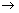(NH4)2SO4

34 g98 g132 g

For 132 g (NH4)2SO4 = 34 g of NH3 is required

So, for 66 g (NH4)2SO4 = 66 32/132 = 17 g of NH3 is required

(b) 17g of NH3 requires volume = 22.4 litres

(c) Mass of acid required, for producing 132g (NH4)2SO4 = 98g

So, Mass of acid required, for 66g (NH4)2SO= 66 98/132 = 49gQuestion 4

The reaction between red lead and hydrochloric acid is given below:

Pb3O+ 8HCl 3PbCl+ 4H2O + Cl2

Calculate: (a) the mass of lead chloride formed by the action of the 6.85 g of red lead, (b) the mass of the chlorine and (c) the volume of the chlorine evolved at S.T.P.Solution 4

(a) Molecular mass of Pb3O= 3 207.2 + 4 16 = 685 g

685 g of Pb3Ogives = 834 g of PbCl2

Hence, 6.85 g of Pb3Owill give = 6.85 834/685 = 8.34 g

(b) 685g of Pb3Ogives = 71g of Cl2

Hence, 6.85 g of Pb3Owill give = 6.85 71/685 = 0.71 g Cl2

(c) 1 V Pb3O4produces 1 V Cl2

685g of Pb3O4has volume = 22.4 litres = volume of Cl2 produced

So, 6.85 Pb3O4 will produce = 6.85 22.4/685 = 0.224 litres of Cl2Question 5

Find the mass of KNO3 required to produce 126 kg of nitric acid. Find whether a larger or smaller mass of NaNO3 is required for the same purpose.

KNO3+ H2SOKHSO4 + HNO3

NaNO+ H2SONaHSO4 + HNO3Solution 5

Molecular mass of KNO= 101 g

63 g of HNO3 is formed by = 101 g of KNO3

So, 126000 g of HNO3 is formed by = 126000 101/63 = 202 kg

Similarly,126 g of HNO3 is formed by 170 kg of NaNO3

So, smaller mass of NaNOis required.Question 6

Pure calcium carbonate and dilute hydrochloric acid are reacted and 2 litres of carbon dioxide was collected at 27oC and normal pressure.

Calculate :

(a) The mass of salt required.

(b) The mass of the acid required to prepare the 2 litres of CO2 at 27 C and normal pressure.

CaCO3 + 2HClCaCl2 + H2O + CO2Solution 6

CaCO3 + 2HClCaCl2 + H2O + CO2

100g73g22.4L

(a)V1 =2 litresV2 =?

T1 = (273+27)=300KT2=273K

V1/T1=V2/T2

V2=V1T2/T1

Now at STP 22.4 litres of CO2 are produced using CaCO3 =100g

So, litres are produced by =100/22.4 2274/300 =.125g

(b)22.4 litres are CO2 are prepared from acid =73g

litres are prepared from = 73/22.4 2273/300=5.9gQuestion 7

Calculate the mass and volume of oxygen at S.T.P., which will be evolved on electrolysis of 1 mole (18g) of waterSolution 7

2H2O2H2 + O2

2 V2 V1 V

2 moles of H2O gives = 1 mole of O2

So, 1 mole of H2O will give = 0.5 moles of O2

so, mass of O2 = no. of moles x molecular mass

= 0.5 32 = 16 g of O2

and 1 mole of O2 occupies volume =22.4 litre

so, 0.5 moles will occupy = 22.4 0.5 = 11.2 litres at S.T.P.Question 8

1.56 g of sodium peroxide reacts with water according to the following equation:

2Na2O2 + 2H2O4NaOH + O2

Calculate:

(a) mass of sodium hydroxide formed,

(b) Volume of oxygen liberated at S.T.P.

(c) Mass of oxygen liberated.Solution 8

2Na2O2 + 2H2O4NaOH + O2

2 V4 V1 V

(a) Mol. Mass of Na2O2 = 2 23 + 2 16 = 78 g

Mass of 2Na2O2= 156 g

156 g Na2Ogives = 160 g of NaOH (4 40 g)

So, 1.56 Na2Owill give = 160 1.56/156 = 1.6 g

(b) 156 g Na2Ogives = 22.4 litres of oxygen

So, 1.56 g will give = 22.4 1.56/156 = 0.224 litres

= 224 cm3

(c)156 g Na2Ogives = 32 g O2

So, 1.56 g Na2Owill give = 32 1.56/156

= 32/100 = 0.32 gQuestion 9

(a) Calculate the mass of ammonia that can be obtained from 21.4 g of NH4Cl by the reaction:

2NH4Cl + Ca(OH)2CaCl2 +2H2O + 2NH3

(b) What will be the volume of ammonia when measured at S.T.P?

The molar volume of a gas = 22.4 litres at STP.Solution 9

2NH4Cl + Ca(OH)2CaCl2+2H2O + 2NH3

2 V1 V1 V2 V

Mol. Mass of 2NH4Cl = 2[14 + (1 4) + 35.5] = 2[53.5] = 107 g

(a) 107 g NH4Cl gives = 34 g NH3

So, 21.4 g NH4Cl will give = 21.4 34/107 = 6.8 g NH3

(b) The volume of 17 g NH3 is 22.4 litre

So, volume of 6.8 g will be = 6.8 22.4/17 = 8.96 litreQuestion 10

Aluminium carbide reacts with water according to the following equation.

Al4C3 + 12H2O → 4Al(OH)3 + 3CH4

(a) What mass of aluminium hydroxide is formed from 12g of aluminium carbide?

(b) What volume of methane s.t.p. is obtained from 12g of aluminium carbide?Solution 10

Question 11

MnO2 + 4HCl MnCl2 + 2H2O +Cl2

0.02 moles of pure MnO2is heated strongly with conc. HCl. Calculate:

(a) mass of MnOused

(b) moles of salt formed,

(c) mass of salt formed,

(d) moles of chlorine gas formed,

(e) mass of chlorine gas formed,

(f) volume of chlorine gas formed at S.T.P.,

(g) moles of acid required,

(h) Mass of acid required.Solution 11

MnO2 + 4HCl MnCl2 + 2H2O +Cl2

1 V4 V1 V1 V

(a) 1 mole of MnO2 weighs = 87 g (mol. Mass)

So, 0.02 mole will weigh = 87 0.02 = 1.74 g MnO2

(b) 1 mole MnO2 gives = 1 mole of MnCl2

So, 0.02 mole MnO2will give =0.02 mole of MnCl2

(c) 1 mole MnCl2 weighs = 126 g(mol mass)

So, 0.02 mole MnCl2 will weigh = 126 0.02 g = 2.52 g

(d) 0.02 mole MnO2will form =0.02 mole of Cl2

(e) 1 mole of Clweighs = 35.5 g

So, 0.02 mole will weigh = 71 0.02 = 1.42 g of Cl2

(f) 1 mole of chlorine gas has volume = 22.4 litres

So, 0.02 mole will have volume = 22.4 0.02 = 0.448 litre

(g) 1 mole MnO2requires HCl = 4 mole

So, 0.02 mole MnO2 will require =4 0.02 = 0.08 mole

(h) For 1 mole MnO, acid required = 4 mole of HCl

So, for 0.02 mole, acid required = 4 0.02 =0.08 mole

Mass of HCl = 0.08 x 36.5 = 2.92 gQuestion 12

Nitrogen and hydrogen react to form ammonia.

N2 (g) + 3H2 (g)2NH3 (g)

If 1000g H2 react with 2000g of N2:

(a) Will any of the two reactants remain unreacted? If yes, which one and what will be its mass?

(b) Calculate the mass of ammonia(NH3) that will be formed?Solution 12

N2 + 3H2 2NH3

28g6g34g

28g of nitrogen requires hydrogen = 6g

2000g of nitrogen requires hydrogen = 6/28 2000=3000/7g

So mass of hydrogen left unreacted =1000-3000/7=571.4g of H2

(b)28g of nitrogen forms NH3 = 34g

2000g of N2 forms NH3

= 34/28 2000

=2428.6g

## Chapter 5 - Mole Concept And Stoichiometry Exercise Misc. Ex.

Question 1

From the equation for burning of hydrogen and oxygen

2H2 + O2 2H2O (Steam)

Write down the number of mole (or moles) of steam obtained from 0.5 moles of oxygen.Solution 1

From equation: 2H2 + O2 2H2O

1 mole of Oxygen gives = 2 moles of steam

so, 0.5 mole oxygen will give = 2 0.5 = 1mole of steamQuestion 2

From the equation

3Cu + 8HNO3 3Cu (NO3)2+ 4H2O + 2NO

(At.mass Cu=64, H=1, N=14,O=16)

Calculate:

(a) Mass of copper needed to react with 63g of HNO3

(b) Volume of nitric oxide at S.T.P. that can be collected.Solution 2

3Cu + 8HNO3 3Cu (NO3)2+ 4H2O + 2NO

1 V8 V3 V2 V

Mol. Mass of 8HNO3 = 8 63 = 504 g

(a) For 504 g HNO3, Cu required is = 192 g

So, for 63g HNO3Cu required = 192 63/504 = 24g

(b) 504 g of HNO3 gives = 2 22.4 litre volume of NO

So, 63g of HNO3 gives =2 22.4 63/504 =5.6 litre of NOQuestion 3

(a) Calculate the number of moles in 7g of nitrogen.

(b) What is the volume at S.T.P. of 7.1 g of chlorine?

(c) What is the mass of 56 cm3 of carbon monoxide at S.T.P?Solution 3

(a) 28g of nitrogen = 1mole

So, 7g of nitrogen = 1/28 7= 0.25 moless

(b) Volume of 71 g of Cl2 at STP =22.4 litres

Volume of 7.1 g chlorine =22.4 7.1/71=2.24 litre

(c) 22400cm3 volume have mass =28 g of CO(molar mass)

So, 56cm3 volume will have mass =28 56/22400= 0.07 gQuestion 4

Some of the fertilizers are sodium nitrate NaNO3, ammonium sulphate (NH4)2SO4 and urea CO(NH2)2. Which of these contains the highest percentage of nitrogen?Solution 4

% of N in NaNO3

% of N in (NH4)2SO4 =

% of N in CO(NH2)2 =

So, highest percentage of N is in urea.Question 5

Water decomposes to O2 and H2 under suitable conditions as represented by the equation below:

2H2O2H2+O2

(a) If 2500 cm3 of H2 is produced, what volume of O2 is liberated at the same time and under the same conditions of temperature and pressure?

(b) The 2500 cm3 of H2 is subjected to 2 times increase in pressure (temp. remaining constant). What volume of H2 will now occupy?

(c) Taking the value of H2 calculated in 5(b), what changes must be made in Kelvin (absolute) temperature to return the volume to 2500 cm3 pressure remaining constant.Solution 5

2H2O2H2+O2

2 V2 V1 V

(a) From equation, 2 V of water gives 2 V of H2 and 1 V of O2

where 2 V = 2500 cm3

so, volume of O2 liberated = 2V/V = 1250 cm3

(b)

(c)

i.e. temperature should be increased by 3.5 times.Question 6

Urea [CO(NH2)2] is an important nitrogeneous fertilizer. Urea is sold in 50 kg sacks. What mass of nitrogen is in one sack of urea?Solution 6

Molecular mass of urea=12 + 16+2(14+2) =60g

60g of urea contains nitrogen =28g

So, in 50g of urea, nitrogen present =23.33 g

50 kg of urea contains nitrogen=23.33kgQuestion 7

Find the molecular formula of a hydrocarbon having vapour density 15, which contains 20% of Hydrogen.Solution 7

% of hydrogen = 20%

% of carbon = 100 - 20 = 80%

Empirical formula = CH3

Empirical formula weight = 1 x 12 + 1 x 3 = 12 + 3 = 15

Vapour Density = 15

Relative molecular mass = 15 x 2 = 30

N =Molecular formula = n x empirical formula

= 2 x CH3

= C2H6Question 8

The following experiment was performed in order to determine the formula of a hydrocarbon. The hydrocarbon X is purified by fractional distillation.

0.145 g of X was heated with dry copper (II) oxide and 224 cm3 of carbon dioxide was collected at S.T.P.

(a) Which elements does X contain?

(b) What was the purpose of copper (II) oxide?

(c ) Calculate the empirical formula of X by the following steps:

(i) Calculate the number of moles of carbon dioxide gas.

(ii) Calculate the mass of carbon contained in this quantity of carbon dioxide and thus the mass of carbon in sample X.

(iii) Calculate the mass of hydrogen in sample X.

(iv) Deduce the ratio of atoms of each element in X (empirical formula).Solution 8

22400cm3 COhas mass = 44g

so, 224 cm3 COwill have mass= 0.44 g

Now since COis being formed and X is a hydrocarbon so it contains C and H.

In 0.44g CO2, mass of carbon=0.44-0.32=0.12g=0.01g atom

So, mass of Hydrogen in X = 0.145-0.12 = 0.025g

= 0.025g atom

Now the ratio of C:H is C=1: H=2.5 or C=2 : H=5

i.e. the formula of hydrocarbon is C2H5

(a) C and H

(b) Copper (II) oxide was used for reduction of the hydrocarbon.

(c)

(i) no. of moles of CO2= 0.44/44 = 0.01 moles

(ii) mass of C = 0.12 g

(iii) mass of H = 0.025 g

(iv) The empirical formula of X = C2H5Question 9

A compound is formed by 24g of X and 64g of oxygen. If atomic mass of X=12 and O=16, calculate the simplest formula of compound.Solution 9

Mass of X in the given compound =24g

Mass of oxygen in the given compound =64g

So total mass of the compound =24+64=88g

% of X in the compound = 24/88 100 = 27.3%

% of oxygen in the compound=64/88 100 =72.7%

Element % At. Mass Atomic ratio Simplest ratio

X 27.3 12 27.3/12=2.27 1

O 72.7 16 72.2/16=4.54 2

So simplest formula = XO2Question 10

A gas cylinder filled with hydrogen holds 5g of the gas. The same cylinder holds 85 g of gas X under same temperature and pressure. Calculate :

(a) Vapour density of gas X.

(b) Molecular weight of gas X.Solution 10

(a) V.D =

(b) Molecular mass = 17(V.D) x 2= 34gQuestion 11

(a) When carbon dioxide is passed over red hot carbon, carbon monoxide is produced according to the equation :

CO2 + C 2CO

What volume of carbon monoxide at S.T.P. can be obtained from 3 g of carbon?

(b) 60 cm3 of oxygen was added to 24 cm3 of carbon monoxide and mixture ignited. Calculate:

(i) volume of oxygen used up and

(ii) Volume of carbon dioxide formed.Solution 11

(a) CO2 + C 2CO

1 V 1 V 2 V

12 g of C gives = 44.8 litre volume of CO

So, 3 g of C will give = 11.2 litre of CO

(b) 2CO + O2 2CO2

2 V 1 V 2 V

(i) 2 V CO requires oxygen = 1 V

so, 24 cm3 CO will require = 24/2 =12 cm3

(ii) 2 x 22400 cm3 CO gives = 2 x 22400 cmCO2

so, 24cm3 CO will give = 24 cmCO2Question 12

How much calcium oxide is obtained by heating 82 g of calcium nitrate? Also find the volume of NO2 evolved:

Solution 12

Molecular weight of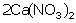=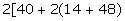=328g

Molecular weight of CaO =2(40+16)

=112g

a. 328g of Ca(NO3)liberates 4 moles of NO2

328g of Ca(NO3)liberatesL of NO82g will liberate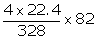=22.4dmof NO2

b. 328 g of calcium nitrate gives 112g of CaO

82 g will give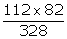=28 g of CaOQuestion 13

The equation for the burning of octane is:

2C8H18 + 25O216CO2 + 18H2O

(i) How many moles of carbon dioxide are produced when one mole of octane burns?

(ii) What volume at S.T.P. is occupied by the number of moles determined in (i)?

(iii) If the relative molecular mass of carbon dioxide is 44, what is the mass of carbon dioxide produced by burning two moles of octane?

(iv) What is the empirical formula of octane?Solution 13

2C8H18 + 25O216CO2 + 18H2O

2 V25 V16 V18 V

(i) 2 moles of octane gives = 16 moles of CO2

so, 1 mole octane will give = 8 moles of CO2

(ii) 1 mole COoccupies volume = 22.4 litre

so, 8 moles will occupy volume = 8 22.4 = 179.2 litre

(iii) 1 mole CO2 has mass = 44 g

so, 16 moles will have mass = 44 16 = 704 g

(iv) Empirical formula is C4H9.Question 14

Ordinary chlorine gas has two isotopes 3517Cl and 3717Cl in the ratio of 3:1. Calculate the relative atomic mass of chlorine.Solution 14

The relative atomic mass of Cl = (35 3 + 1 37)/4=35.5 amuQuestion 15

Silicon (Si = 28) forms a compound with chlorine (Cl = 35.5) in which 5.6 g of silicon combines with 21.3 g of chlorine. Calculate the empirical formula of the compound.Solution 15

Mass of silicon in the given compound =5.6g

Mass of the chlorine in the given compound=21.3g

Total mass of the compound=5.6g+21.3g=26.9g

% of silicon in the compound = 56/26.9 100 = 20.82%

% of chlorine in the compound = 21.2/26.9 100 = 79.18%

Element % At. Mass At. Ratio Simplest ratio

Si 20.82 28 20.82/28=0.74 1

Cl 79.18 35.5 79.18/35.5=2.23 3

So the empirical formula of the given compound =SiCl3Question 16

An acid of phoshorus has the following percentage composition; Phosphorus = 38.27%; hydrogen = 2.47 %; oxygen = 59.26 %. Find the empirical formula of the acid and its molecular formula, given that its relative molecular mass is 162.Solution 16

% composition Atomic ratio Simple ratio

P = 38.27% 38.27/31 =1.23 1

H = 2.47% 2.47/1 = 2.47 2

O = 59.26% 59.26/16 = 3.70 3

So, empirical formula is PH2O3 or H2PO3

Empirical formula mass = 31+ 2 1 + 3 16 = 81

The molecular formula is = H4P2O6, because n = 162/81=2Question 17

a) Calculate the mass of substance 'A' which in gaseous form occupies 10 litres at 270C and 700 mm pressure. The molecular mass of 'A' is 60.

b) A gas occupied 360 cm3 at 870C and 380 mm Hg pressure. If the mass of gas is 0.546 g, find its relative molecular mass.Solution 17

a) V1 = 10 litres V2=?

T1= 27+ 273 = 300KT2=273K

P1=700 mmP2 = 760 mm

Using the gas equation

b)

Question 18

A gas cylinder can hold 1 kg of hydrogen at room temperature and pressure.

(a) What mass of carbon dioxide can it hold under similar conditions of temperature and pressure?

(b) If the number of molecules of hydrogen in the cylinder is X, calculate the number of carbon dioxide molecules in the cylinder. State the law that helped you to arrive at the above resultSolution 18

(a) Molecular mass of CO2 = 12+ 2x16 = 44 g

So, vapour density (V.D) = mol. Mass/2 = 44/2 = 22

V.D =

So, mass of CO2 = 22 kg

(b) According to Avogadros law ,equal volumes of all gases under similar conditions of temperature and pressure contain equal number of molecules.

So, number of molecules of carbon dioxide in the cylinder =number of molecules of hydrogen in the cylinder=XQuestion 19

Following questions refer to one mole of chlorine gas.

(a) What is the volume occupied by this gas at S.T.P.?

(b) What will happen to volume of gas, if pressure is doubled?

(c) What volume will it occupy at 2730C?

(d) If the relative atomic mass of chlorine is 35.5, what will be the mass of 1 mole of chlorine gas?Solution 19

(a) The volume occupied by 1 mole of chlorine = 22.4 litre

(b) Since PV=constant so, if pressure is doubled; the volume will become half i.e. 11.2 litres.

(c) V1/V2 = T1/T2

22.4/V2 =273/546

V2 = 44.8 litres

(d) Mass of 1 mole Cl2 gas =35.5 x 2 =71 gQuestion 20

(a) A hydrate of calcium sulphate CaSO4.xH2O contains 21% water of crystallisation. Find the value of x.

(b) What volume of hydrogen and oxygen measured at S.T.P. will be required to prepare 1.8 g of water.

(c) How much volume will be occupied by 2g of dry oxygen at 270C and 740 mm pressure?

(d) What would be the mass of CO2 occupying a volume of 44 litres at 250 C and 750 mm pressure?

(e) 1 g of a mixture of sodium chloride and sodium nitrate is dissolved in water. On adding silver nitrate solution, 1.435 g of AgCl is precipitated.

AgNO3 (aq) + NaCl (aq) AgCl (s) + NaNO3

Calculate the precentage of NaCl in the mixture.Solution 20

(a) Total molar mass of hydrated CaSO4.xH2O = 136+18x

Since 21% is water of crystallization, so

So, x = 2 i.e. water of crystallization is 2.

(b) For 18 g water, vol. of hydrogen needed = 22.4 litre

So, for 1.8 g, vol. of H2 needed= 1.8 x 22.4/18 = 2.24 litre

Now 2 vols. of water = 1 vol. of oxygen

1 vol. of water =1/2 vol. of O2 =22.4/2=11.2 lit.

18 g of water = 11.2 lit. of O2

1.8 g of water = 11.2/18 18/10=1.12 lit.

(c) 32g of dry oxygen at STP = 22400cc

2g will occupy = 224002/32=1400cc

P1=760mm P2 =740mm

V1=1400cc V2 =?

T1 =273 K, T2 = 27 +73 = 300K

(d) P1= 750mm P2=760mm

V1= 44lit. V2=?

T1= 298K T2=273K

(e) Since 143.5g of AgCl is produced from =58.5 g of NaCl

so, 1.435 g of AgCl is formed by =0.585 g of NaCl

% of NaCl =0.585 x100 = 58.5%Question 21

a. From the equation :

Calculate :

i. The mass of carbon oxidized by 49 g of sulphuric acid.

ii. The volume of sulphur dioxide measured at STP,  liberated at the same time.

b.

i. A compound has the following percentage composition by mass: carbon 14.4%, hydrogen 1.2% and chlorine 84.5%. Determine the empirical formula of this compound. Work correct to 1 decimal place. (H = 1; C = 12; Cl = 35.5)

ii. The relative molecular mass of this compound is 168, so what is its molecular formula?Solution 21

a.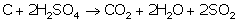For CO12+32

i. Molecular mass of sulphuric acid = 2(2+32+64)

= 196

196 g of suphuric acid oxidized 12g of Carbon

49 g of suphuric acid will=3 g

ii. 196 g of sulphuric acid gives 2(22.4)

=44.8L49 g og sulphuric acid will give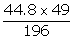=11.2 L of SO2

b.

i.

Empirical formula = CHCl2

ii.

Empirical formula = CHCl2

Empirical formula weight = 1 x 12 + 1 x 1+(2 x35.5)

= 12 + 1+70

= 83

Relative molecular mass = 168

N =2.02≈2

Molecular formula = n x empirical formula

= 2 x CHCl2

= C2H2Cl4Question 22

Find the percentage of

a. oxygen in magnesium nitrate crystals [Mg (NO3) 6H2O].

b. boron in Na2B4O7.10H2O. [H = 1, B = 11, O =16, Na = 23].

c. phosphorus in the fertilizer superphosphate Ca(H2PO4)2Solution 22

a. Relative molecular mass of [Mg (NO3) 6H2O]

=24+14+(3 x16)+(6 x18)=194

Since, 194g of [Mg (NO3) 6H2O] contains 144g of oxygen100g of [Mg (NO3) 6H2O] containsof oxygen = 74.22%

b. Relative molecular mass of Na2B4O7.10H2O

(23 × 2) + (4 × 11) + (7 × 16) + 10(18) = 382

Since 382g of Na2B4O7.10H2O contains 44g of boron

100g Na2B4O7.10H2O of contains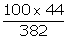of boron

=11.5%

c. Relative molecular mass of Ca(H2PO4)2

= 40 + 2(2 + 31 + 64) = 234

Since,234g of Ca(H2PO4)2 contains 62g of phosphorus

100g of Ca(H2PO4)2 contains=26.5%Question 24

Solid ammonium dichromate decomposes as:

If 63 g of ammonium dichromate decomposes. Calculate

a. the quantity in moles of (NH4)2Cr2O7

b. the quantity in moles of nitrogen formed

c. the volume of N2 evolved at STP.

d. what will be the loss of mass ?

e. calculate the mass of chromium (III) oxide formed at the same time.Solution 24

Solid ammonium dichromate decomposes as:

(a)Molecular mass of ammonium dichromate

= 2(14+4)+104+112

= 252 g

Number of moles=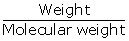==0.25moles

(b)

252 g of ammonium dichromate gives 22.4 dm3 of N2

63 g of ammonium dichromate gives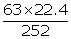=5.6 L

= 0.25 moles

(c)

252 g of ammonium dichromate gives 22.4 dm3 of N2

63 g of ammonium dichromate gives= 5.6 L

(d)

Number of moles=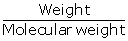==0.25moles

0.25 moles of ammonium dichromate gives

0.25 moles of N2=7 g

1 mole of H2O =18 g

Therefore, total loss of mass=7+18

=25 g

(e)

252 g of ammonium dichromate gives 152 g of CrO3

63 g of ammonium dichromate gives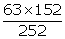=38 gQuestion 25

Hydrogen sulphide gas burns in oxygen to yield 12.8 g of sulphur dioxide gas as under :

2H2S + 3O2 2H2O + 2SO2

Calculate the volume of hydrogen sulphide at S.T.P. Also, calculate the volume of oxygen required at S.T.P. which will complete the combustion of hydrogen sulphide determined in (litres).Solution 25

2H2S + 3O2 2H2O + 2SO2

2 V 3 V 2 V

128 g of SO2 gives = 2 22.4 litres volume

So, 12.8 g of SO2 gives = 2 22.4 12.8/128

= 4.48 litre volume

Or one can say 4.48 litres of hydrogen sulphide.

22.4 litre H2S requires oxygen = 3 22.4 litre

So, 4.48 litres H2S will require = 6.72 litre of oxygenQuestion 26

Ammonia burns in oxygen and the combustion, in the presence of a catalyst. May be represented by 2NH3 + 2O2 ? 2NO + 3H2O

[H= 1, N= 14, O=16]

What mass of steam is produced when 1.5 g of nitrogen monoxide is formed?Solution 26

From equation, 2NH3 + 2 O2 2NO + 3H2O

When 60 g NO is formed, mass of steam produced = 54 g

So, 1.5 g NO is formed, mass of steam produced = 54 1.5/60

=1.35 gQuestion 27

If a crop of wheat removes 20 kg of nitrogen per hectare of soil, what mass of the fertilizer, calcium nitrate Ca(NO3)2 would be required to replace the nitrogen in a 10 hectare field ?Solution 27

In 1 hectare of soil, N2 removed = 20 kg

So, in 10 hectare N2 removed = 200 kg

The molecular mass of Ca(NO3)2 =164

Now, 28 g Npresent in fertilizer = 164 g Ca(NO3)2

So, 200000 g of N2 is present in = 164 200000/28

= 1171.42 kgQuestion 28

Concentrated nitric acid oxidises phosphorus to phosphoric acid according to the following equation:

P+ 5HNO3 H3PO4+ 5NO2 + H2O

If 6.2g of phosphorus was used in the reaction calculate:

(a) Number of moles of phosphorus taken and mass of phosphoric acid formed.

(b) mass of nitric acid will be consumed at the same time?

(c) The volume of steam produced at the same time if measured at 760 mm Hg pressure and 2730C?Solution 28

(a) 1 mole of phosphorus atom = 31 g of phosphorus

31 g of P =1 mole of P

6.2g of P = =0.2 mole of P

(b) 31 g P reacts with HNO= 315 g

so, 6.2 g P will react with HNO3 = 315 6.2/31 = 63 g

(c)

Moles of steam formed from 31g phosphorus = 18g/18g = 1mol

Moles of steam formed from 6.2 g phosphorus = 1mol/31g6.2=0.2 mol

Volume of steam produced at STP =0.2 22.4 l/MOL=4.48 litre

Since the pressure (760mm) remains constant , but the temperature (273+273)=546 is double, the volume of the steam also gets doubled

So,Volume of steam produced at 760mm Hg and 2730C=4.48 2=8.96litreQuestion 29

112 cm3 of a gaseous fluoride of phosphorus has a mass of 0.63 g. Calculate the relative molecular mass of the fluoride. If the molecule of the fluoride contains only one atom of phosphorus, then determine the formula of the phosphorus fluoride. [ F=19, P=31].Solution 29

112cmof gaseous fluoride has mass = 0.63 g

so, 22400cm3 will have mass = 0.63 22400/112

= 126 g

The molecular mass = At mass P + At. mass of F

126= 31 + At. Mass of F

So, At. Mass of F = 95 g

But, at. mass of F = 19 so 95/19 = 5

Hence, there are 5 atoms of F so the molecular formula = PF5Question 30

Washing soda has formula Na2CO3.10H2O. What mass of anhydrous sodium carbonate is left when all the water of crystallization is expelled by heating 57.2 g of washing soda?Solution 30

Na2CO3.10H2Na2CO3 + 10H2O

286 g 106 g

So, for 57.2 g Na2CO3.10H2O = 106 57.2/286 = 21.2 g Na2CO3Question 31

A metal M forms a volatile chloride containing 65.5% chlorine. If the density of the chloride relative to hydrogen is 162.5, find the molecular formula of the chloride (M = 56).Solution 31

Simple ratio of M = 34.5/56 = 0.616 = 1

Simple ratio of Cl = 65.5/35.5 = 1.845 = 3

Empirical formula = MCl3

Empirical formula mass = 162.5, Molecular mass = 2 V.D = 325

So, n = 2

So, molecular formula = M2Cl6Question 32

A compound X consists of 4.8% carbon and 95.2% bromine by mass.

(i) Determine the empirical formula of this compound working correct to one decimal place (C = 12 ; Br = 80)

(ii) If the vapour density of the compound is 252, what is the molecular formula of the compound?Solution 32

(i) Element%atomic massatomic ratiosimple ratio

C4.8121

Br95.2803

So, empirical formula is CBr3

(ii) Empirical formula mass = 12 + 3 80 = 252 g

molecular formula mass = 2 252(V.D) = 504 g

n= 504/252 = 2

so, molecular formula = C2Br6Question 33

The reaction: 4N2O + CH4 CO2 + 2H2O + 4N2 takes place in the gaseous state. If all volumes are measured at the same temperature and pressure, calculate the volume of dinitrogen oxide (N2O) required to give 150 cm3 of steam.Solution 33

4N2O + CH4 CO2 + 2H2O + 4N2

4 V 1 V 1 V 2 V 4 V

2 x 22400 litre steam is produced by N2O = 4 x 22400 cm3

So, 150 cm3 steam will be produced by= 4 22400 150/2 x 22400

= 300 cmN2OQuestion 34

Samples of the gases O2, N2, CO2 and CO under the same conditions of temperature and pressure contain the same number of molecules x. The molecules of oxygen occupy V litres and have a mass of 8 g under the same of temperature and pressure

What is the volume occupied by:

(a) x molecules of N2

(b) 3x molecules of CO

(c) What is the mass of CO2 in grams?

(d) In answering the above questions, which law have you used?Solution 34

(a) Volume of O2 = V

Since Oand Nhave same no. of molecules = x

so, the volume of N= V

(b) 3x molecules means 3V volume of CO

(c) 32 g oxygen is contained in = 44 g of CO2

So, 8 g oxygen is contained in = 44 x 8/32 = 11 g

(d) Avogadro's law is used in the above questions.Question 35

The percentage composition of sodium phosphate as determined by analysis is 42.1% sodium, 18.9% phosphorus and 39% oxygen. Find the empirical formula of the compound?Solution 35

simple ratio of Na = 42.1/23 = 1.83 = 3

simple ratio of P = 18.9/31 = 0.609 = 1

simple ratio of O = 39/16 = 2.43 = 4

So, the empirical formula is Na3PO4Question 36

What volume of oxygen is required to burn completely a mixture of 22.4 dm3 of methane and 11.2 dm3 of hydrogen into carbon dioxide and steam?

CH4 + 2O2 CO2 + 2H2O

2H2 + O2 2H2OSolution 36

CH4 + 2O2 CO2 + 2H2O

1 V 2 V 1 V 2 V

From equation:

22.4 litres of methane requires oxygen = 44.8 litres O2

2H2 + O2 2H2O

2 V 1 V 2 V

From equation,

44.8 litres hydrogen requires oxygen = 22.4 litres O2

So, 11.2 litres will require = 22.4 x 11.2/44.8 = 5.6 litres

Total volume = 44.8 + 5.6 = 50.4 litresQuestion 37

The gases hydrogen, oxygen, carbon dioxide, sulphur dioxide and chlorine are arranged in order of their increasing relative molecular masses. Given 8 g of each gas at S.T.P., which gas will contain the least number of molecules and which gas the most?Solution 37

Equal volumes of all gases, under similar conditions of temperature and pressure ,contain equal number of molecules.

So, 1 mole of each gas contains = 6.02 1023 molecules

Mol. Mass of H2 (2),O2(32) ,CO2(44),SO2(64),Cl2(71)

(1)Now 2 g of hydrogen contains molecules =6.02 1023

So, 8g of hydrogen contains molecules = 8/2 6.02 1023

=4 6.02 1023 = 4M molecules

(2)32g of oxygen contains molecules = 8/32 6.02 1023=M/4

(3)44g of carbon dioxide contains molecules = 8/44 6.02 1023=2M/11

(4)64g of sulphur dioxide contains molecules =6.02 1023

So, 8g of sulphur dioxide molecules = 8/64 6.02 1023= M/8

(5)71 g of chlorine contains molecules =6.02 1023

So, 8g of chlorine molecules = 8/72 6.02 1023 = 8M/71

Since 8M/71<M/8<2M/11<M/4<4M

Thus Cl2<SO2<CO2<O2<H2

(i)Least number of molecules in Cl2

(ii)Most number of molecules in H2Question 38

10 g of a mixture of sodium chloride and anhydrous sodium sulphate is dissolved in water. An excess of barium chloride solution is added and 6.99 g of barium sulphate is precipitated according to the equation given below:

Na2SO4 + BaCl2 BaSO4 + 2NaCl

Calculate the percentage of sodium sulphate in the original mixture.Solution 38

Na2SO4 + BaCl2 BaSO4 + 2NaCl

Molecular mass of BaSO= 233 g

Now, 233 g of BaSOis produced by Na2SO= 142 g

So, 6.99 g BaSOwill be produced by = 6.99 142/233 = 4.26

The percentage of Na2SOin original mixture = 4.26 100/10

= 42.6%Question 39

When heated, potassium permanganate decomposes according to the following equation :

(a) Some potassium permanganate was heated in the test tube. After collecting one litre of oxygen at room temperature, it was found that the test tube had undergone a loss in mass of 1.32 g. If one litre of hydrogen under the same conditions of temperature and pressure has a mass of 0.0825 g, calculate the relative molecular mass of oxygen.

(b) Given that the molecular mass of potassium permanganate is 158. What volume of oxygen (measured at room temperature) would be obtained by the complete decomposition of 15.8 g of potassium permanganate? (Molar volume at room temperature is 24 litres)Solution 39

(a) 1 litre of oxygen has mass = 1.32 g

So, 24 litres (molar vol. at room temp.) will have mass = 1.32 x 24

= 31.6 or 32 g

(b) 2KMnO4 K2MnO4 + MnO2 + O2

316 g of KMnO4 gives oxygen = 24 litres

So, 15.8 g of KMnOwill give = 24 316/15.8 = 1.2 litresQuestion 40

(a) A flask contains 3.2 g of sulphur dioxide. Calculate the following:

(i) The moles of sulphur dioxide present in the flask.

(ii) The number of molecules of sulphur dioxide present in the flask.

(iii) The volume occupied by 3.2 g of sulphur dioxide at S.T.P.

(S= 32, O= 16)

(b) An Experiment showed that in a lead chloride solution, 6.21 g of lead is combined with 4.26 g of chlorine. What is the empirical formula of this chlorine? (Pb = 207; Cl = 35.5)Solution 40

(a)

(i) The no. of moles of SO2 = 3.2/64 = 0.05 moles

(ii) In 1 mole of SO2, no. of molecules present = 6.02 1023

So, in 0.05 moles, no. of molecules = 6.02 1023 0.05

= 3.0 1022

(iii) The volume occupied by 64 g of SO2 = 22.4 dm3

3.2 g of SO2 will be occupied by volume = 22.4 3.2/64 =1.12 dm3

(b) Gram atoms of Pb = 6.21/207=0.03 = 1

Gram atoms of Cl = 4.26/35.5 = 0.12 = 4

So, the empirical formula = PbCl4Question 41

The volume of gases A, B, C and D are in the ratio, 1 : 2 : 2 : 4 under the same conditions of temperature and pressure .

(i) Which sample of gas contains the maximum number of molecules?

(ii) If the temperature and pressure of gas A are kept constant, then what will happen to the volume of A when the number of molecules is doubled?

(iii)If this ratio of gas volume refers to the reactants and products of a reaction, which gas law is being observed?

(iv)If the volume of A is actually 5.6 dm3 at S.T.P., calculate the number of molecules in the actual Volume of D at S.T.P. (Avogadro's number is 6 1023).

(v) Using your answer from (iv), state the mass of D if the gas is dinitrogen oxide (N2O)Solution 41

(i) D contains the maximum number of molecules because volume is directly proportional to the number of molecules.

(ii) The volume will become double because volume is directly proportional to the no. of molecules at constant temperature and pressure.

V1/V2 = n1/n2

V1/V2 = n1/2n1

So, V= 2V1

(iii) Gay lussac's law of combining volume is being observed.

(iv) The volume of D = 5.6 4 = 22.4 dm3, so the number of molecules = 6 x 1023 because according to mole concept 22.4 litre volume at STP has = 6 x 10 23 molecules

(v) No. of moles of D = 1 because volume is 22.4 litre

so, mass of N2O = 1 44 = 44 gQuestion 42

The equation given below relate the manufacture of sodium carbonate (molecular weight of Na2CO3 = 106).

1. NaCl+NH3+ CO+ H2ONaHCO3+NH4Cl

2. 2NaHCO3 Na2CO3+H2O + CO2

Equations (1) and (2) are based on the production of 21.2 g of sodium carbonate.

(a) What mass of sodium hydrogen carbonate must be heated to give 21.2 g of sodium carbonate?

(b) To produce the mass of sodium hydrogen carbonate calculate in (a), what volume of carbon dioxide, measured at S.T.P. would be required?Solution 42

(a) NaCl+NH3+ CO+ H2ONaHCO3+NH4Cl

2NaHCO3 Na2CO3+H2O + CO2

From equation:

106 g of Na2COis produced by = 168 g of NaHCO3

So, 21.2 g of Na2COwill be produced by = 168 21.2/106

= 33.6 g of NaHCO3

(b) For 84 g of NaHCO3, requiredvolume of CO2 = 22.4 litre

So, for 33.6 g of NaHCO3, required volume of CO2 = 22.4 x 33.6/84

= 8.96 litreQuestion 43

A sample of ammonium nitrate when heated yields 8.96 litres of steam (measure at S.T.P.)

NH4NO3N2O + 2H2O

(i) What volume of dinitrogen oxide is produced at the same time as 8.96 litres of steam?

(ii) What mass of ammonium nitrate should be heated to produce 8.96 litres of steam? (Relative molecular mass of ammonium nitrate is 80)

(iii) Determine the percentage of oxygen in ammonium nitrate (O = 16).Solution 43

(a) NH4NO3N2O+2H2O

1mole1mole2mole

1 V1 V2 V

44.8 litres of water produced by = 22.4 litres of NH4NO3

So, 8.96 litres will be produced by = 22.4 x 8.96/44.8

= 4.48 litres of NH4NO3

So, 4.48 litres of N2O is produced.

(i) 44.8 litre H2O is produced by = 80 g of NH4NO3

So, 8.96 litre H2O will be produced by = 80 x 8.96/44.8

= 16g NH4NO3

(iii) % of O in NH4NO3 = 3x16/80 = 60%Question 44

Given that the relative molecular mass of copper oxide is 80, what volume of ammonia (measured at STP) is required to completely reduce 120g of copper oxide? The equation for the reaction is :

Solution 44

Molecular mass of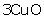=(240) g

Molecular mass of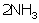= 2 × 22.4 = 44.8dm3

Molecular mass of= (192)g

240 g of CuO requires 44.8 dmof NH3120g of CuO will require=22.4dm3Question 45

(a) Calculate the number of moles and the number of molecules present in 1.4 g of ethylene gas. What is the volume occupied by the same amount of ethylene?

(b) What is the vapour density of ethylene?Solution 45

(a) The molecular mass of ethylene(C2H4) is 28 g

No. of moles = 1.4/28 = 0.05 moles

No. of molecules = 6.023 x1023 x 0.05 = 3 x 1022 molecules

Volume = 22.4 x 0.05 = 1.12 litres

(b) Molecular mass = 2 X V.D

S0, V.D = 28/2 = 14Question 46

(a) Calculate the percentage of sodium in sodium aluminium fluoride (Na3AlF6) correct to the nearest whole number.

(F = 19; Na =23; Al = 27)

(b) 560 ml of carbon monoxide is mixed with 500 ml of oxygen and ignited. The chemical equation for the reaction is as follows:

2CO + O2 2CO2

Calculate the volume of oxygen used and carbon dioxide formed in the above reaction.Solution 46

(a) Molecular mass of Na3AlF= 210

So, Percentage of Na = 3x23x100/210 = 32.85%

(b) 2CO + O2 2CO2

2 V 1 V 2 V

1 mole of O2 has volume = 22400 ml

Volume of oxygen used by 2 x 22400 ml CO = 22400 ml

So, Vol. of O2 used by 560 ml CO =22400 x 560/(2 x 22400)

= 280 ml

So, Volume of CO2 formed is 560 ml.Question 47

a. A gas cylinder of capacity of 20 dmis filled with gas X the mass of which is 10 g. When the same cylinder is filled with hydrogen gas at the same temperature and pressure the mass of the hydrogen is 2 g, hence the relative molecular mass of the gas is:

i. 5

ii. 10

iii. 15

iv. 20

b.

i. Calcium carbide is used for the artificial ripening of fruits. Actually the fruit ripens because of the heat evolved while calcium carbide reacts with moisture. During this reaction calcium hydroxide and acetylene gas is formed. If 200 cm3 of acetylene is formed from a certain mass of calcium carbide, find the volume of oxygen required and carbon dioxide formed during the complete combustion. The combustion reaction can be represented as below.

ii. A gaseous compound of nitrogen and hydrogen contains 12.5% hydrogen by mass. Find molecular formula of the compound if its relative molecular mass is 37. [N = 14, H = 1].

c.

i. A gas cylinder contains 24 x 1024 molecules of nitrogen gas. If Avogadro's number is 6 x 1023 and the relative atomic mass of nitrogen is 14, calculate :

1. Mass of nitrogen gas in the cylinder

2. Volume of nitrogen at STP in dm3.

(ii) Commercial sodium hydroxide weighing 30g has some sodium chloride in it. The Mixture on dissolving in water and subsequent treatment with excess silver nitrate solution formed a precipitate weighing 14.3 g. What is the percentage of sodium chloride in the commercial sample of sodium hydroxide? The equation for the reaction is

[Relative molecular mass of NaCl = 58; AgCl = 143]

(iii) A certain gas 'X' occupies a volume of 100 cm3 at S.T.P. and weighs 0.5 g. Find its relative molecular massSolution 47

a. Mass of gas X =10g

Mass of hydrogen gas= 2

Relative vapour density

=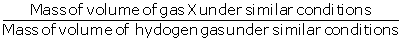==5

Relative molecular mass of the gas= 2×relative vapour

density = 2×5

=10

b.

i. The combustion reaction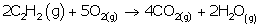According to Gay-Lussac's law,

2 volume of acetylene requires 5 volume of oxygen to burn it1 volume of acetylene requires 2.5 volume of oxygen to burn it200cmrequires 2.5×200=500 cmof oxygen

2 volume of acetylene on combustion gives 4CO1 volume of acetylene on combustion gives 2CO200cc of acetylene on combustion will give  200×2=400cc of CO2

ii. Hydrogen = 12.5%

∴ Nitrogen= 100-12.5= 87.5%

The Empirical formula of the compound is NH2

Empirical formula weight =14+2=16

Relative molecular mass =37

N =2.3≈2

Molecular formula = n x empirical formula  = 2 x NH2

=N2H4

c.

i. Molecules of nitrogen gas in a cylinder = 24 x 1024

Avogadro's number = 6 x 1023

1. Mass of nitrogen in a cylinder  =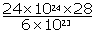=1120g

2. Volume of nitrogen at stp

Volume of 28 g of N2  = 22.4dm

Volume of 1120g of N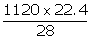dm3

=896 dm3Question 48

a.

i. LPG stands for liquefied petroleum gas. Varieties of LPG are marketed including a mixture of propane (60%) and butane (40%). If 10 litre of this mixture is burnt, find the total volume of carbon dioxide gas added to the atmosphere. Combustion reactions can be represented as :

s

ii. Calculate the percentage of nitrogen and oxygen in ammonium nitrate. [Relative molecular mass of ammonium nitrate is 80, H = 1, N = 14, O = 16].

b. 4.5 moles of calcium carbonate are reacted with dilute hydrochloric acid.

i. Write the equation for the reaction.

ii. What is the mass of 4.5 moles of calcium carbonate? (Relative molecular mass of calcium carbonate is 100)

iii. What is the volume of carbon dioxide liberated at STP?

iv. What mass of calcium chloride is formed? (Relative molecular mass of calcium chloride is 111).

v. How many moles of HCl are used in this reaction?Solution 48

a.

i. 10 litres of LPG contains

Propane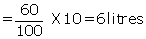Butane

18+16=34 L

ii. Molecular mass of NH4(NO3) =80

H=1, N=14, O=16

% of Nitrogen

As 80 g of NH4(NO3) contains 28 g of nitrogen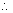100 g of of NH4(NO3) will contain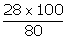= 35%

% of Oxygen

As,80 g of NH4(NO3) contains 48 g of oxygen100 g of of NH4(NO3) will contain= 60%

b.

i. Equation for reaction of calcium carbonate with dilute hydrochloric acid:

ii. Relative molecular mass of calcium carbonate=100

Mass of 4.5 moles of calcium carbonate

= No. of moles× Relative molecular mass

= 4.5×100

= 450g

iii.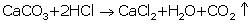As, 100g of calcium carbonate gives 22.4dm3 of CO2450 g of calcium carbonate will give=100.8 L

iii. Molecular mass of calcium carbonate =100

Relative molecular mass of calcium chloride =111

As 100 g of calcium carbonate gives 111g of calcium chloride450 g of calcium carbonate will give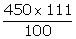=499.5 g

iv.

Molecular mass of HCl=36.5

Molecular mass of calcium carbonate =100

As 100 g of calcium carbonate gives (2×36.5)= 73g of HCl450 g of calcium carbonate will give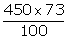=328.5g

Number of moles of HCl=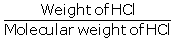=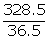= 9 molesQuestion 49

a.

i. Calculate the volume of 320 g of SO2 at STP. (Atomic mass: S = 32 and O = 16).

ii. State Gay-Lussac's Law of combining volumes.

iii. Calculate the volume of oxygen required for the complete combustion of 8.8 g of propane (C3H8). (Atomic mass: C = 12, O = 16, H = I, Molar Volume = 22.4 dm3 at stp.)

b.

i. An organic compound with vapour density = 94 contains C = 12.67%, H = 2.13%, and Br = 85.11%. Find the molecular formula. [Atomic mass : C = 12, H = 1, Br = 80]

ii. Calculate the mass of:

1. 1022 atoms of sulphur.

2. 0.1 mole of carbon dioxide.

[Atomic mass : S = 32, C = 12 and O = 16 and

Avogadro's Number = 6 x 1023]Solution 49

a.

i. Atomic mass: S = 32 and O = 16

Molecular mass of SO2=32+(2×16)

=64g

As 64 g of SO2 = 22.4dm3

Then, 320 g of SO2 =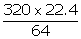=112 L

ii. Gay-Lussac's law Gay-Lussac's Law states "When gases react, they do so in volumes which bear a simple ratio to one another and to the volume of the gaseous product, if all the volumes are measured at the same temperature and pressure."

iii. C3H8 + 5O2→3CO2 + 4H2O

Molar mass of propane = 44

44 g of propane requires 5 × 22.4 litres of oxygen at STP.

8.8 g of propane requires= 22.4 litres

b.

i.

Empirical formula = CH2Br

n(Empirical formula mass of CH2Br) = Molecular mass (2 × VD)

n(12 + 2 + 80) = 94 × 2

n = 2

Molecular formula = Empirical formula × 2

= (CH2Br) × 2

= C2H4Br2

ii. 1022 atoms of sulphur

6.022 × 1023 atoms of sulphur will have mass = 32 g

1022 atoms of sulphur will have mass =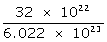= 0.533 g

iii. 0.1 mole of carbon dioxide

1 mole of carbon dioxide will have mass = 44 g

0.1 mole of carbon dioxide will have mass = 4.4 gQuestion 50

a. Concentrated nitric acid oxidises phosphorus to phosphoric acid according to the following equation:

If 9.3 g of phosphorus was used in the reaction, calculate :

i. Number of moles of phosphorus taken.

ii. The mass of phosphoric acid formed.

iii. The volume of nitrogen dioxide produced at STP.

b.

i. 67.2 litres of hydrogen combines with 44.8 litres of nitrogen to form ammonia under specific conditions as:

Calculate the volume of ammonia produced. What is the other substance, if any, that remains in the resultant mixture?

ii. The mass of 5.6 dm3 of a certain gas at STP is 12.0 g. Calculate the relative molecular mass of the gas.

iii. Find the total percentage of Magnesium in magnesium nitrate crystals, Mg(NO3)2-6H2O.

[Mg = 24, N = 14, 0 = 16 and H = 1]Solution 50

a.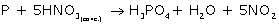i. Number of moles of phosphorus taken =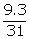= 0.3 mol

ii. 1 mole of phosphorus gives 98 gm of phosphoric  acid.

So, 0.3 mole of phosphorus gives (0.3 × 98) gm of  phosphoric acid

= 29.4 gm of phosphoric acid

iii. 1 mole of phosphorus gives 112 L of NO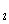gas at STP.

So, 0.3 mole of phosphorus gives (112 × 0.3) L of

NOgas at STP.

= 33.6 L of NOgas at STP

b.

i. According to the equation

3 volumes of hydrogen produce 2 volumes of ammonia

67.2 litres of hydrogen produce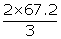= 44.8 L

3 volumes of hydrogen combine with 1 volume of ammonia.

67.2 litres of hydrogen combine with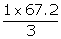=22.4L Nitrogen left = 44.8 - 22.4 = 22.4 litres

ii. 5.6 dm3 of gas weighs 12 g

1 dm3 of gas weighs = (12/56) gm

22.4 dm3 of gas weighs = (12/56 × 22.2) gm = 48g

Therefore, the relative molecular mass of gas = 48 gm.

iii. Molar mass of Mg (NO3)2.6H2O

= 24 × (14 × 2) + (16 × 12) + (1 × 12) = 256 g

Mass percent of magnesium =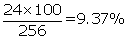Question 51

a.

i. What volume of oxygen is required to burn completely 90 dm3 of butane under similar conditions of temperature and pressure?

ii. The vapour density of a gas is 8. What would be the volume occupied by 24.0 g of the gas at STP?

iii. A vessel contains X number of molecules of hydrogen gas at a certain temperature and pressure. How many molecules of nitrogen gas would be present in the same vessel under the same conditions of temperature and pressure?

b. O2 is evolved by heating KClO3 using MnO2 as a catalyst.

i. Calculate the mass of KClO3 required to produce 6.72 litre of O2 at STP.

[atomic masses of K = 39, Cl = 35.5, 0 = 16].

ii. Calculate the number of moles of oxygen present in the above volume and also the number of molecules.

iii. Calculate the volume occupied by 0.01 mole of CO2 at STP.Solution 51

a.

i.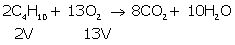2 vols. of butane requires O2 = 13 vols

90 dm3 of butane will require O2 =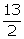× 90

= 585 dm3

ii. Molecular mass = 2 × Vapour density

So, molecular mass of gas = 2 × 8 = 16 g

As we know, molecular mass or molar mass occupies 22.4 litres.

That is,

16 g of gas occupies volume = 22.4 litres

So, 24 g of gas will occupy volume

=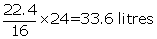iii. According to Avogadro's law, equal volumes of all gases under similar conditions of temperature and pressure contain the same number of molecules.

So, molecules of nitrogen gas present in the same vessel = X

b.

i.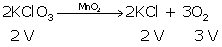3 vols. of oxygen require KClO3 = 2 vols.

So, 1 vol. of oxygen will require KClO3 =So, 6.72 litres of oxygen will require KClO3

=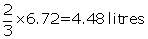22.4 litres of KClO3 has mass = 122.5 g

So, 4.48 litres of KClO3 will have mass

=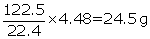ii. 22.4 litres of oxygen = 1 mole

So, 6.72 litres of oxygen =No. of molecules present in 1 mole of O2

= 6.023 × 1023

So, no. of molecules present in 0.3 mole of O2

= 6.023 × 1023 × 0.3

= 1.806 × 1023

iii. Volume occupied by 1 mole of CO2 at STP = 22.4  litres

So, volume occupied by 0.01 mole of CO2 at STP = 22.4 × 0.01= 0.224 litresQuestion 52

a.

i. Oxygen oxidises ethyne to carbon dioxide and water as shown by the equation:

What volume of ethyne gas at s.t.p. is require to produce 8.4 dm3 of carbon dioxide at STP [H = 1, C = 12, 0 = 16]

ii. A compound made up of two elements X and Y has an empirical formula X2Y. If the atomic weight of X is 10 and that of Y is 5 and the compound has a vapour density (V.D.) 25, find its molecular formula.

b. A cylinder contains 68 g of Ammonia gas at STP

i. What is the volume occupied by this gas?

ii. How many moles of ammonia are present in the cylinder?

iii. How many molecules of ammonia are present in the cylinder?Solution 52

a.

i.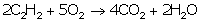2 moles of C2H2=4moles of CO2

x dm3 of C2H =8.4 dm3 of CO2

x==4.2 dm3 of C2H

ii. Empirical formula= X2Y

Atomic weight (X)= 10

Atomic weight (Y)= 5

Empirical formula weight = (2 × 10) + 5

=25

= 2

So, molecular formula = X2Y×2

= X4Y

b.

i. A cylinder contains 68 g of ammonia gas at STP.

Molecular weight of ammonia = 17 g/mole

68 g of ammonia gas at STP =?

1 mole = 22.4 dm3

∴ 4 mole = 22.4 × 4 = 89.6 dm3

ii. 4 moles of ammonia gas is present in the cylinder.

iii. 1 mole = 6.023 × 1023 molecules

4 moles = 24.092 × 1023 molecules

error: Content is protected !!